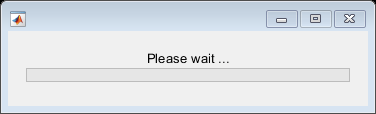afterAll

Run function after all functions finish running in the background

Description

example

B = afterAll(A,fcn,n) returns a Future object B and runs the function fcn automatically after all elements in the Future array A finish.

MATLAB® runs the function fcn using the concatenated outputs from each element in A. The outputs Y1,...,Ym from each Future object are concatenated vertically, in linear index order. For example, if A is a two-element Future vector with outputs y1 and y2 from the first and second Future objects respectively, MATLAB runs fcn([y1; y2]) after all elements in A finish.

If the Future array A has M elements, MATLAB runs the function only once. When the scheduled function fcn finishes, the Future object B finishes.

You create a Future object when:

• You run a function in the background using backgroundPool.

• You run a function on a parallel pool when you have Parallel Computing Toolbox™.

For more information about using afterAll in parallel, see Use afterEach and afterAll to Run Callback Functions (Parallel Computing Toolbox).

If any of the elements in A encounters an error, the Error property of B is an MException.

B = afterAll(A,fcn,n,PassFuture=true) runs fcn(A) instead of using the concatenated outputs of each element in A.

The Error property of B is an empty MException array, even if one or more elements in A encounter an error.

Examples

collapse all

This example shows how to use afterEach to update a wait bar with the progress of functions running in the background.

Create a wait bar, w.Set the number of iterations for your for-loop, N. Store the current number of completed iterations, 0, and the total number of iterations, N, in the UserData property of the wait bar.

N =20;
w.UserData = [0 N];

Run a for-loop with N iterations. In each iteration, use parfeval and backgroundPool to run pause in the background for a random number of seconds. Store each Future object in an array.

for i = 1:N
delay = rand;
f(i) = parfeval(backgroundPool,@pause,0,delay);
end

Use the helper function updateWaitbar to update the waitbar after each Future finishes.

afterEach(f,@(~)updateWaitbar(w),0);

Use delete to close the wait bar after all the Future objects finish.

afterAll(f,@(~)delete(w),0);

Define Helper Function

Define the helper function updateWaitbar. The function increments the first element of the UserData property, then uses the vector to calculate the progress.

function updateWaitbar(w)
% Update a waitbar using the UserData property.

% Check if the waitbar is a reference to a deleted object
if isvalid(w)
% Increment the number of completed iterations
w.UserData(1) = w.UserData(1) + 1;

% Calculate the progress
progress = w.UserData(1) / w.UserData(2);

% Update the waitbar
waitbar(progress,w);
end
end

Input Arguments

collapse all

Input Future object, specified as a parallel.Future scalar or array.

MATLAB runs the function fcn after each element in A finishes. If any of the elements in A encounters an error and you specify PassFuture as true, afterAll still runs fcn using that element.

• If you specify PassFuture as true, the function is run as fcn(Aj) using each Future element Aj in A. If any of the elements in A encounters an error, afterAll does not finish with an error.

• Otherwise, the function is run as fcn(X1,...,Xm) using the outputs X1,...,Xm from each Future element in A. If any of the elements in A encounters an error, afterAll finishes with an error.

If the Future array has M elements, MATLAB runs the function M times. When the scheduled function fcn finishes, the Future object B finishes.

Example: A = parfeval(backgroundPool,@magic,1,3);

Callback function to run, specified as a function handle.

Example: fcn = @magic

Number of output arguments, specified as a nonnegative integer scalar.

• If you specify PassFuture as true, n is the number of output arguments expected from running fcn(A) using the Future array A.

• Otherwise, n is the number of output arguments expected from running fcn(Y1,...,Ym) using the vertically concatenated outputs Y1,...,Ym from each element Aj in the Future array A.

Data Types: single | double | int8 | int16 | int32 | int64 | uint8 | uint16 | uint32 | uint64

Output Arguments

collapse all

Output Future object, returned as a parallel.Future object.

• Use fetchOutputs to retrieve results from B.

• Use afterEach or afterAll to run a function when B completes.

When you set PassFuture, you change the Error property of B:

• If PassFuture is true, the Error property of B is an empty MException array, even if one or more elements in A encounter an error.

• Otherwise, if any of the elements in A encounters an error, the Error property of B is an MException object.

To find the cause of the error, use the cause property of B.Error.